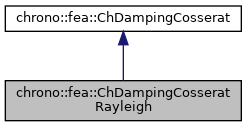chrono::fea::ChDampingCosseratRayleigh Class Reference

## Description

Simple Rayleigh damping of beam sections of Cosserat type, where damping is proportional to stiffness via a beta coefficient.

In order to generalize it also in case of nonlinearity, the full element tangent stiffness matrix cannot be used (it may contain negative eigenvalues) and it can't be used to recover instant nodal caused by damping as F=beta*K*q_dt so it is generalized to the following implementation at the material stress level

  {n,m}=beta*[E]*{e',k'}


where

• beta is the 2nd Rayleigh damping parameter
• [E] is the 6x6 material stiffness matrix at the undeformed unstressed case (hence assumed constant)
• {e',k'} is the speed of deformation/curvature Note that the alpha mass-proportional parameter (the first of the alpha,beta parameters of the original Rayleigh model) is not supported.

#include <ChBeamSectionCosserat.h>

Inheritance diagram for chrono::fea::ChDampingCosseratRayleigh:[legend]
Collaboration diagram for chrono::fea::ChDampingCosseratRayleigh:[legend]

## Public Member Functions

ChDampingCosseratRayleigh (std::shared_ptr< ChElasticityCosserat > melasticity, const double &mbeta=0)
Construct the Rayleigh damping model from the stiffness model used by the section. More...

virtual void ComputeStress (ChVector<> &stress_n, ChVector<> &stress_m, const ChVector<> &dstrain_e, const ChVector<> &dstrain_k) override
Compute the generalized cut force and cut torque, caused by structural damping, given actual deformation speed and curvature speed. More...

virtual void ComputeDampingMatrix (ChMatrixNM< double, 6, 6 > &R, const ChVector<> &dstrain_e, const ChVector<> &dstrain_k) override
Compute the 6x6 tangent material damping matrix, ie the jacobian [Rm]=dstress/dstrainspeed. More...

double GetBeta ()
Get the beta Rayleigh parameter (stiffness proportional damping)

void SetBeta (const double mbeta)
Set the beta Rayleigh parameter (stiffness proportional damping)

After you added this damping to a ChBeamSectionCosserat, in case you have changed some parameters in the stiffness model after creating this Rayleigh damping, you must call this UpdateStiffnessModel() method so that here we update the [E] 6x6 material stiffness matrix, which is stored here as private and constant data for performance.Public Attributes inherited from chrono::fea::ChDampingCosserat
ChBeamSectionCosseratsection

## ◆ ChDampingCosseratRayleigh()

 chrono::fea::ChDampingCosseratRayleigh::ChDampingCosseratRayleigh ( std::shared_ptr< ChElasticityCosserat > melasticity, const double & mbeta = 0 )

Construct the Rayleigh damping model from the stiffness model used by the section.

This is important because the Rayleigh damping is proportional to the stiffness, so the model must know which is the stiffness matrix of the material. Note: melasticity must be alreay set with proper values: its [E] stiffness matrix will be fetched just once for all.

## ◆ ComputeDampingMatrix()

 void chrono::fea::ChDampingCosseratRayleigh::ComputeDampingMatrix ( ChMatrixNM< double, 6, 6 > & R, const ChVector<> & dstrain_e, const ChVector<> & dstrain_k )
overridevirtual

Compute the 6x6 tangent material damping matrix, ie the jacobian [Rm]=dstress/dstrainspeed.

In this model, it is beta*[E] where [E] is the 6x6 stiffness matrix at material level, assumed constant

Parameters
 R 6x6 material stiffness matrix values here dstrain_e current strain speed (deformation part) dstrain_k current strain speed (curvature part)

Reimplemented from chrono::fea::ChDampingCosserat.

## ◆ ComputeStress()

 void chrono::fea::ChDampingCosseratRayleigh::ComputeStress ( ChVector<> & stress_n, ChVector<> & stress_m, const ChVector<> & dstrain_e, const ChVector<> & dstrain_k )
overridevirtual

Compute the generalized cut force and cut torque, caused by structural damping, given actual deformation speed and curvature speed.

Parameters
 stress_n local stress (generalized force), x component = traction along beam stress_m local stress (generalized torque), x component = torsion torque along beam dstrain_e local strain speed (deformation); x elongation speed; y,z shear speeds dstrain_k local strain speed (curvature); x torsion speed; y,z line curvature speeds

Implements chrono::fea::ChDampingCosserat.

The documentation for this class was generated from the following files:
• /builds/uwsbel/chrono/src/chrono/fea/ChBeamSectionCosserat.h
• /builds/uwsbel/chrono/src/chrono/fea/ChBeamSectionCosserat.cpp